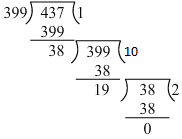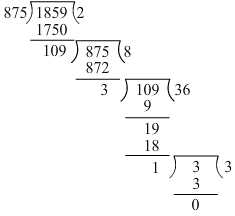Courses

# RD Sharma Solutions: Exercise 2.6- Playing With Numbers Class 6 Notes | EduRev

## Class 6 : RD Sharma Solutions: Exercise 2.6- Playing With Numbers Class 6 Notes | EduRev

The document RD Sharma Solutions: Exercise 2.6- Playing With Numbers Class 6 Notes | EduRev is a part of the Class 6 Course Mathematics (Maths) Class 6.
All you need of Class 6 at this link: Class 6

Q.1. Determine the H.C.F of the following numbers by using Euclid's algorithm (i-x):

(i) 300,450

(ii) 399,437

(iii) 1045,1520

Ans:

(i) 300 and 450

Dividend = 450 and divisor = 300Clearly, the last divisor is 150.

Hence, HCF of the given numbers is 150.

(ii) 399 and 437

We have dividend = 399 and divisor = 437Clearly, the last divisor is 19.

Hence, HCF of the given numbers is 19.

(iii) 1045 and 1520

We have dividend = 1045 and divisor = 1520Clearly, the last divisor is 95.

Hence, HCF of the given numbers is 95.

Q.2. Show that the following pairs are co-prime:

(i) 59,97

(ii) 875,1859

(iii) 288,1375

Ans: We know that two numbers are co-primes if their HCF is 1.

(i) 59 and 97

Here, dividend = 97 and divisor = 59Clearly, the last divisor is 1.

Hence, the given numbers are co-primes.

(ii) 875 and 1859

Here, dividend = 1,859 and divisor = 875Clearly, the last divisor is 1.

Hence, the given numbers are co-primes.

(iii) 288 and 1375

Here, dividend = 288 and divisor = 1,375Clearly, the last divisor is 1.

Hence, the given numbers are co-primes.

Q.3. What is the H.C.F of two consecutive numbers?

Ans:

The HCF of two consecutive numbers is 1.

Example:

D = 4 and d = 5 are two consecutive numbers.

Here, we have dividend = 5 and divisor = 4Clearly, the last divisor is 1.

Hence, HCF of 4 and 5 is 1.

Q.4. Write true (T) of false (F) for each of the following statements:

(i) The H.C.F of two distinct prime numbers is 1.

(ii) The H.C.F of two co-prime number is 1.

(iii) The H.C.F of an even and an odd numbers is 1.

(iv) The H.C.F of two consecutive even numbers is 2.

(v) The H.C.F of two consecutive odd numbers is 2.

Ans:

(i) True.

(ii) True.

(iii) False.

HCF of 6 and 9 is 3, not 1.

(iv) True.

(v) False.

HCF of two consecutive odd number is 1.

Example: HCF of 25 and 27 is 1.

Offer running on EduRev: Apply code STAYHOME200 to get INR 200 off on our premium plan EduRev Infinity!

## Mathematics (Maths) Class 6

191 videos|224 docs|43 tests

,

,

,

,

,

,

,

,

,

,

,

,

,

,

,

,

,

,

,

,

,

;